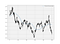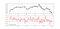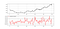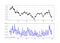# The Equilibrium Indicator — Profiting from Mean-Reversion.

## Coding and Trading the Reversion to Equilibrium in Python.

Markets swing between extremes and move in cyclical phases. Sometimes, they are a bit overbought due to a superior optimistic feeling and other times they are oversold due to looming fear. The self-similarity and fractal natures of the financial markets tell us that this goes for all the time frames. In this article, we will see how to profit from fading extreme moves using the Equilibrium method.

If you are interested by market sentiment and how to model the sentiment of institutional traders, feel free to have a look at the below article:

# The Concept of Moving Averages

Moving averages help us confirm and ride the trend. They are the most known technical indicator and this is because of their simplicity and their proven track record of adding value to the analyses. We can use them to find support and resistance levels, stops and targets, and to understand the underlying trend. This versatility makes them an indispensable tool in our trading arsenal.EURUSD hourly values with the 200-period simple moving average.

As the name suggests, this is your plain simple mean that is used everywhere in statistics and basically any other part in our lives. It is simply the total values of the observations divided by the number of observations. Mathematically speaking, it can be written down as:

We can see that the moving average is providing decent dynamic support and resistance levels from where we can place our orders in case the market goes down there.

The code for the moving average can be written down as the following:

`def ma(Data, lookback, what, where):      for i in range(len(Data)):      try:        Data[i, where] = (Data[i - lookback + 1:i + 1, what].mean())                    except IndexError:                pass    return Data`

To use it, we need to have an OHLC data array with an extra empty column. This can be done by using the following code:

`# Defining the function that adds a certain number of columnsdef adder(Data, times):        for i in range(1, times + 1):            z = np.zeros((len(Data), 1), dtype = float)        Data = np.append(Data, z, axis = 1)    return Data# Adding 1 extra columnmy_data = adder(my_data, 1)# Calling the moving average functionmy_data = ma(my_data, 200, 3, 4)`

The above states that the moving average function will be called on the array named my_data for a lookback period of 200, on the column indexed at 3 (closing prices in an OHLC array). The moving average values will then be put in the column indexed at 4 which is the one we have added using the adder function.USDCHF hourly values with the 200-period simple moving average.

# The Equilibrium Indicator

The state of Equilibrium can be measured in many complex ways ranging from advanced mathematics to complex computations, but the way I see it is, why complicate things? We can create a very simple proxy to measure the market’s position relative to its implied Equilibrium. By implied, we mean that it can be an average that the market often reverts to. This is usually a short-term moving average where the price frequently closes above and below it.

This means that this short-term moving average is not like the ones we use to find dynamic support and resistance levels, but one that can be used to tell us that the market is likely to go towards its direction.

We will measure Equilibrium as being the exponentially smoothed average of the distance between the market price and its moving average.

This means that we will follow the below steps:

• Calculate a simple 5-period moving average of the market price.
• Subtract the current market price from its moving average.
• Calculate a 5-period exponential moving average on the subtracted values.

The result is the 5-period Equilibrium Indicator that we will use to generate mean-reverting signals.EURUSD in the first panel with the 5-period Equilibrium Indicator.

The above plot shows an example on the EURUSD hourly data plotted with the Equilibrium Indicator in blue. The full code can be find below:

`def ema(Data, alpha, lookback, what, where):        # alpha is the smoothing factor    # window is the lookback period    # what is the column that needs to have its average calculated    # where is where to put the exponential moving average        alpha = alpha / (lookback + 1.0)    beta  = 1 - alpha        # First value is a simple SMA    Data = ma(Data, lookback, what, where)        # Calculating first EMA    Data[lookback + 1, where] = (Data[lookback + 1, what] * alpha) + (Data[lookback, where] * beta)    # Calculating the rest of EMA    for i in range(lookback + 2, len(Data)):            try:                Data[i, where] = (Data[i, what] * alpha) + (Data[i - 1, where] * beta)                    except IndexError:                pass    return Datadef deleter(Data, index, times):        for i in range(1, times + 1):            Data = np.delete(Data, index, axis = 1)return Data   def jump(Data, jump):        Data = Data[jump:, ]        return Datadef equilibrium(Data, lookback, close, where):        # Calculating the moving average    Data = ma(Data, lookback, close, where)    Data = jump(Data, lookback)        # Calculating the distance from the moving average    Data[:, where + 1] = Data[:, close] - Data[:, where]        # Calculating the Exponential Moving Average of the Equilibrium    Data = ema(Data, 2, lookback, where + 1, where + 2)    Data = jump(Data, lookback)    Data = deleter(Data, where, 2)        return Data# Using the functionmy_data = equilibrium(Data, 5, 3, 4)`USDCHF in the first panel with the 5-period Equilibrium Indicator.

If you are also interested by more technical indicators and using Python to create strategies, then my latest book may interest you.:

# Back-testing the Strategy

As with any proper research method, the aim is to back-test the indicator and to be able to see for ourselves whether it is worth having as an add-on to our pre-existing trading framework. Note that the below only back-tests one time frame on only 10 currency pairs for the last 10 years. It is possible that this is not the optimal time frame for the strategy, but we are just trying to find a one-shoe-size-almost-fits-all strategy.

The conditions are as expected:

• Go long (Buy) whenever the 5-period Equilibrium Indicator reaches -0.001 with the two previous values greater than -0.001. Hold this position until getting a new signal (where the position is closed) or getting stopped out by the ATR risk management system.
• Go short (Sell) whenever the Percentile Range Indicator reaches 0.001 with the two previous values below 0.001. Hold this position until getting a new signal (where the position is closed) or getting stopped out by the ATR risk management system.

The below shows the signal function used to generate the trades:

`def signal(Data, what, buy, sell):        for i in range(len(Data)):                    if Data[i, what] < lower_barrier and Data[i - 1, what] > lower_barrier and Data[i - 2, what] > lower_barrier :            Data[i, buy] = 1                    if Data[i, what] > upper_barrier and Data[i - 1, what] < upper_barrier and Data[i - 2, what] < upper_barrier :            Data[i, sell] = -1# Using the function assuming the extra columns have been addedsignal(my_data, 4, 5, 6)`

Now, when we apply the strategy on ten major currency pairs using the hourly data with a 0.5 spread per transaction cost and a 0.20 theoretical risk-reward ratio. We obtain the following.

The results below are encouraging but as I always say, they are not enough to form a solid part of a systematic strategy. This is because of the low transaction fees I have used that are generally very hard to obtain and also the fact that it assumes no slippage. This can be corrected by the use of limit orders but only a highly specialized expert can obtain the fees and code the strategy with a respectable broker. At the moment, our job is to find predictive strategies and know how to filter the good from the bad ones.

# A Word on Risk Management

When I say I use ATR-based risk management system (Average True Range), it means that the algorithm will do the following steps with regards to the position it takes.

A long (Buy) position:

• The algorithm initiates a buy order after a signal has been generated following a certain strategy.
• Then, the algorithm will monitor the ticks and whenever the high equals a certain constant multiplied by ATR value at the time of the trade inception, an exit (at profit) order is initiated. Simultaneously, if a low equals a certain constant multiplied by ATR value at the time of the trade inception is seen, an exit (at loss) is initiated. The exit encountered first is naturally the taken event.

A short (Sell) position:

• The algorithm initiates a short sell order after a signal has been generated following a certain strategy.
• Then, the algorithm will monitor the ticks and whenever the low equals a certain constant multiplied by ATR value at the time of the trade inception, an exit (at profit) order is initiated. Simultaneously, if a high equals a certain constant multiplied by ATR value at the time of the trade inception is seen, an exit (at loss) is initiated. The exit encountered first is naturally the taken event.EURUSD with the 10-period eATR.

The plot above shows the Average True Range I generally use. It is based on an exponential moving average as opposed to the original smoothed moving average.

Take a look at the latest value on the ATR. It is around 0.0014 (14 pips). If we initiate a buy order following a simple 2.00 risk-reward ratio (risking half of what we expect to gain), we can place an order this way:

• Buy at current market price.
• Take profit at current market price + (2 x 14 pips).
• Stop the position at current market price — (1 x 14 pips).

The code I use for the Average True Range indicator is as follows:

`def ema(Data, alpha, lookback, what, where):        # alpha is the smoothing factor    # window is the lookback period    # what is the column that needs to have its average calculated    # where is where to put the exponential moving average        alpha = alpha / (lookback + 1.0)    beta  = 1 - alpha        # First value is a simple SMA    Data = ma(Data, lookback, what, where)        # Calculating first EMA    Data[lookback + 1, where] = (Data[lookback + 1, what] * alpha) + (Data[lookback, where] * beta)        # Calculating the rest of EMA    for i in range(lookback + 2, len(Data)):            try:                Data[i, where] = (Data[i, what] * alpha) + (Data[i - 1, where] * beta)                    except IndexError:                pass    return Datadef eATR(Data, lookback, high, low, close, where):        # TR    for i in range(len(Data)):        try:                        Data[i, where] = max(Data[i, high] - Data[i, low],                               abs(Data[i, high] - Data[i - 1, close]),                               abs(Data[i, low] - Data[i - 1, close]))                    except ValueError:            pass            Data[0, where] = 0        Data = ema(Data, 2, lookback, where, where + 1)        return Data`

# Conclusion

If you regularly follow my articles, you will find that many of the indicators I develop or optimize have a high hit ratio and on average are profitable. This is mostly due to the risk management method I use. But what about market randomness and the fact that many underperformers blaming Technical Analysis for their failure?

First of all, I constantly publish my trading logs on Twitter before initiation and after initiation to show the results. This ensures transparency. I also publish a track record on Twitter every 1–3 months. However, I never guarantee a return nor superior skill whatsoever. As for the indicators that I develop, I constantly use them in my personal trading. Hence, I have no motive to publish biased research. My goal is to share back what I have learnt from the online community.

Remember to always do your back-tests. Even though I supply the indicator’s function (as opposed to just brag about it and say it is the holy grail and its function is a secret), you should always believe that other people are wrong. My indicators and style of trading works for me but maybe not for everybody. I rely on this rule:

The market price cannot be predicted or is very hard to be predicted more than 50% of the time. But market reactions can be predicted.

What the above quote means is that we can form a small zone around an area and say with some degree of confidence that the market price will show a reaction around that area. But we cannot really say that it will go down 4% from there, then test it again, and breakout on the third attempt to go to \$103.85. The error term becomes exponentially higher because we are predicting over predictions.

While we are discussing this topic, I should point out a few things about my back-tests and articles:

• The spread I use is based on institutional quotes of a small pip fraction. Generally, retail traders are given a whopping spread of 1–2 pips per trade. This is huge and unfair to them. I use 0.2–0.5 spread. However, most of the strategies that use the hourly time frame still work with 1 pip spread. For the ones that use M15 or M5 time frames, they cannot be profitable with a spread of 1 pip.
• The holding period calculation I use is close-to-close in case there is no risk management process.
• Although I discourage trading based on just one indicator, the numbers do not lie. What I am presenting is what could have happened when taking into account a low spread.
• Some of the back-tests I provide are losers and they are published either to demystify a trading myth or to present interesting functions to be coded by readers.
• Finally, I am a firm believer of not spoon-feeding the learners. I have learnt by doing and not by copying. You should get the idea, the function, the intuition, the conditions of the strategy, and then elaborate (an even better) one yourself so that you back-test it and improve it before deciding to take it live or to eliminate it.

To sum up, are the strategies I provide realistic? Yes, but with optimizing the environment (robust algorithm, low costs, honest broker, risk management). Are the strategies provided only for the sole use of trading? No, it is to stimulate brainstorming and getting more trading ideas as we are all sick of hearing about an oversold RSI as a reason to go short or a resistance being surpassed as a reason to go long.

## Sign up for Geek Culture Hits

### By Geek Culture

Subscribe to receive top 10 most read stories of Geek Culture — delivered straight into your inbox, once a week. Take a look.

By signing up, you will create a Medium account if you don’t already have one. Review our Privacy Policy for more information about our privacy practices.

Check your inbox
Medium sent you an email at to complete your subscription.

Written by

## Sofien Kaabar

Institutional FOREX Strategist & Trader. Author of “The Book of Back-tests” https://www.amazon.com/dp/B089CWQWF8

## Geek Culture

A new tech publication by Start it up (https://medium.com/swlh).

Written by

## Sofien Kaabar

Institutional FOREX Strategist & Trader. Author of “The Book of Back-tests” https://www.amazon.com/dp/B089CWQWF8## Geek Culture

A new tech publication by Start it up (https://medium.com/swlh).

## More From Medium

Medium is an open platform where 170 million readers come to find insightful and dynamic thinking. Here, expert and undiscovered voices alike dive into the heart of any topic and bring new ideas to the surface. Learn more

Follow the writers, publications, and topics that matter to you, and you’ll see them on your homepage and in your inbox. Explore

If you have a story to tell, knowledge to share, or a perspective to offer — welcome home. It’s easy and free to post your thinking on any topic. Write on Medium